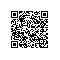# java基础学习5_方法重构

public class JavaDemo{
public static void main(String args[]){
int resultA = sum(10,20);
int resultB = sum(10,20,30);
double resultC = sum(10.2,30.3);
System.out.println("加法执行结果为：" + resultA);
System.out.println("加法执行结果为：" + resultB);
System.out.println("加法执行结果为：" + resultC);
}

public static int sum(int x,int y){
return x + y;
}
public static int sum(int x,int y,int z){
return x + y + z;
}
public static double sum(double x,double y){
return x + y;
}
}

public class JavaDemo{
public static void main(String args[]){
System.out.println(1);
System.out.println(1.2);
System.out.println(true);
System.out.println('真');
System.out.println("hello world");
}
}

System.out.println本身就属于一个方法重载